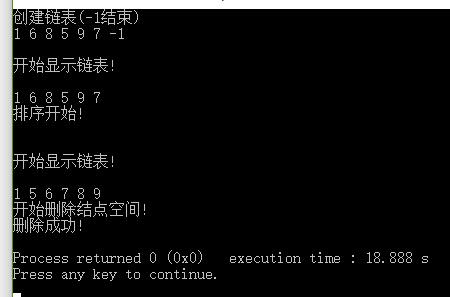2016-09-29 11:59

# c++ 数据结构 单链表问题

• 点赞
• 写回答
• 关注问题
• 收藏
• 邀请回答

#### 2条回答默认 最新

•凝视深空 2016-09-29 15:11
已采纳

#include
using namespace std;

typedef struct node
{
int data;
struct node *next;
}list;

void swap(int *a,int *b)
{
int t=*a;
*a=*b;
*b=t;
}

{
cout<<"创建链表(-1结束)\n";
list *p,*q;
while(1)
{
q=new list;
cin>>q->data;
if(q->data==-1)
{
q=NULL;
p->next=q;
break;
}
else
p->next=q;
p=q;
}
cout<<endl;
}

{
cout<<"排序开始!\n\n";
list *p,*q,*r;
for(p=r;p->next!=NULL;p=p->next)
for(q=p->next;q!=NULL;q=q->next)
if(p->data>q->data)
swap(&q->data,&p->data);
cout<<endl;
}

{
cout<<"开始显示链表!\n\n";
list *p;
while(p)
{
cout<data<<" ";
p=p->next;
}
cout<<endl;
}

{
cout<<"开始删除结点空间!\n";
list *p,*q;
while(p)
{
q=p->next;
delete p;
p=q;
}
cout<<"删除成功!\n";
}

int main(void)
{
•